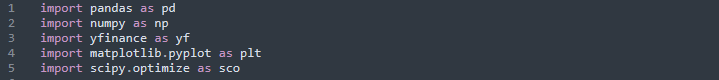Home Python Trading Strategy Mean-Variance Portfolio In Python: A Practical Guide

# Mean-Variance Portfolio In Python: A Practical Guide

This article explores the implementation of a mean-variance portfolio in Python. It delves into the core concepts of Modern Portfolio Theory in Section 1 and proceeds to provide a practical Python example in Section 2. Modern Portfolio Theory is a significant methodology widely applied in financial investment, focusing on optimizing returns while minimizing risk through diversification. This article demonstrates the process of constructing an optimal portfolio using mathematical equations, plotting financial series, and solving for the efficient frontier.

### Key Takeaways:

1. The article discusses the implementation of a mean-variance portfolio in Python using Modern Portfolio Theory.
2. Modern Portfolio Theory focuses on maximizing returns while minimizing risk through diversification.
3. The article explains how to construct a portfolio, estimate mean and covariance, and generate 10,000 random portfolios.
4. Portfolio optimization aims to find the optimal weights to achieve the minimum variance for a given target return.
5. The efficient frontier is plotted to visualize the trade-off between risk and return in the portfolio.

## Introduction

This article will implement a mean-variance portfolio in Python. Section 1 will discuss the main concepts of the Modern Portfolio Theory; subsequently, Section 2 will implement the practical part with a Python example.

## Modern Portfolio Theory

This theory is one of the most relevant methodologies in financial investment; many financial institutions have applied it across the financial market. When an investor opens an account with a robo-advisor or an investment management firm, the trading firm will ask you about your risk profile (risk tolerance); the intention is to construct a portfolio following the Modern Portfolio Theory guidelines. This example is one of the multiple cases.

The goal of any investor is to maximize the returns and keep the risk level as low as possible through diversification. Many possible allocation combinations exist to build a portfolio; however, the objective is to find the minimum risk, giving a predefined return.

## Optimal Portfolio Illustration

The following example shows three investments, two with 10% and one with 15% return, respectively. Each investment has two financial instruments, stocks A and B, with different weights.

The first step to identify the optimal portfolio is to set the level of return, in this case is 10%. Portfolio 3 has 15% and does not follow the criteria; therefore, we will keep Portfolio A and B. Next is to find the portfolio with the minimum volatility; hence, Portfolio 1 with 5% is the optimal portfolio.

## Construction Portfolio

The returns to construct a portfolio follow a multivariate normal distribution:

## Plotting the Financial Series in Python

These are the Python libraries to use:The financial instruments:

The code to download the data and get the closing prices from the previous securities is:

In any time series analysis, it is important to plot the historical evolution. The libraries pandas and matplotlib will do the trick:

The plot associated with the previous image is:

In the previous chart, it is difficult to compare the historical evolution of the closing price among different financial instruments because the starting price is different between them. It is convenient to make the following transformation:

The chart is:

The historical prices tend to have an upward trend over time (this is also one of the reasons we like to trade stocks).

## Mean and Variance Estimation

Following the section Construction Portfolio, we need to estimate the mean and covariance. The first step is to transform the returns from daily to yearly (data_return = data_ret * 252) and also the covariance (data_covariance = data_ret.cov() * 252):

Generation of 10,000 Random Portfolios

In this section, we will generate and plot 10,000 random portfolios. The scope is to see the risk-return structure in a chart.

First, we create two Python lists (returns_list and volatility_list) to append the return and volatility for each random portfolio. We make random weights inside the loop and transform them to ensure that the total investment is 100% (normalized_weights). Then, the portfolio return (rpreturn) is calculated using the inner product (). Finally, we calculate the random volatility (rpstd) using. The code is:

The following image shows the Python procedure to plot the 10,000 random portfolios:

We multiply the pandas data frame by 100 to get the values in percentage. The plot associated with the previous image is:

This chart reveals a half-elliptical curve pattern. If we set as our target return 25%, there are too many portfolios.

## Portfolio Optimization

In this part, we will solve the optimization problem in equation 3. The output will be the optimal weights that generate the portfolio with the minimum variance, giving a target level of return.

We need to make two functions to calculate the mean and variance:

The implementation of the solver minimize from scipy (sco.minimize) is:

The goal in (sco.minimize) is to minimize the function that calculates the volatility (std_portfolio) subject to the restrictions and bounds already commented.

The next table shows that BRK-B has 71% and the remaining assets in total less than 29%.

Let’s see how the previous portfolio performs against an equal-weighted portfolio:

## Plotting the Efficient Frontier

This section will plot the efficient frontier. Combining the code from the two previous two images, we have:

In the code, it is essential to mention that we will generate an efficient frontier with 150 values. Next, it follows the estimation of the target return ii/50. Subsequently, it follows the constraints and the minimization process. The remaining coding line is a reimplementation of previous sections.

The plot is: